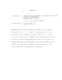## Measurement of Z Boson Transverse Momentum In Proton-Antiproton Collisions At sqrt(s)=1.96 TeV##### Files
umi-umd-4193.pdf(3.11 MB)
This dissertation describes a measurement of the shape of the boson transverse momentum distribution in $p\bar{p}\rightarrow Z/\gamma^{*}\rightarrow e^+e^- +X$ events at a center-of-mass energy of 1.96 TeV. The measurement is made for events with electron-positron mass between $70 < M_{ee} < 110$ GeV/c$^{2}$ and uses 976 pb$^{-1}$ of data collected at the Fermilab Tevatron collider with the D\O\ detector. The shape is measured both for the inclusive sample and for the subset of events containing a boson with large rapidity. The large-rapidity distribution shows better agreement with theory when the calculation is done using traditional Collins-Soper-Sterman resummation than when using a recent resummed form factor with modifications in the small-x region.